Statement questions - Sum of n terms

Chapter 5 Class 10 Arithmetic Progressions (Term 2)
Concept wise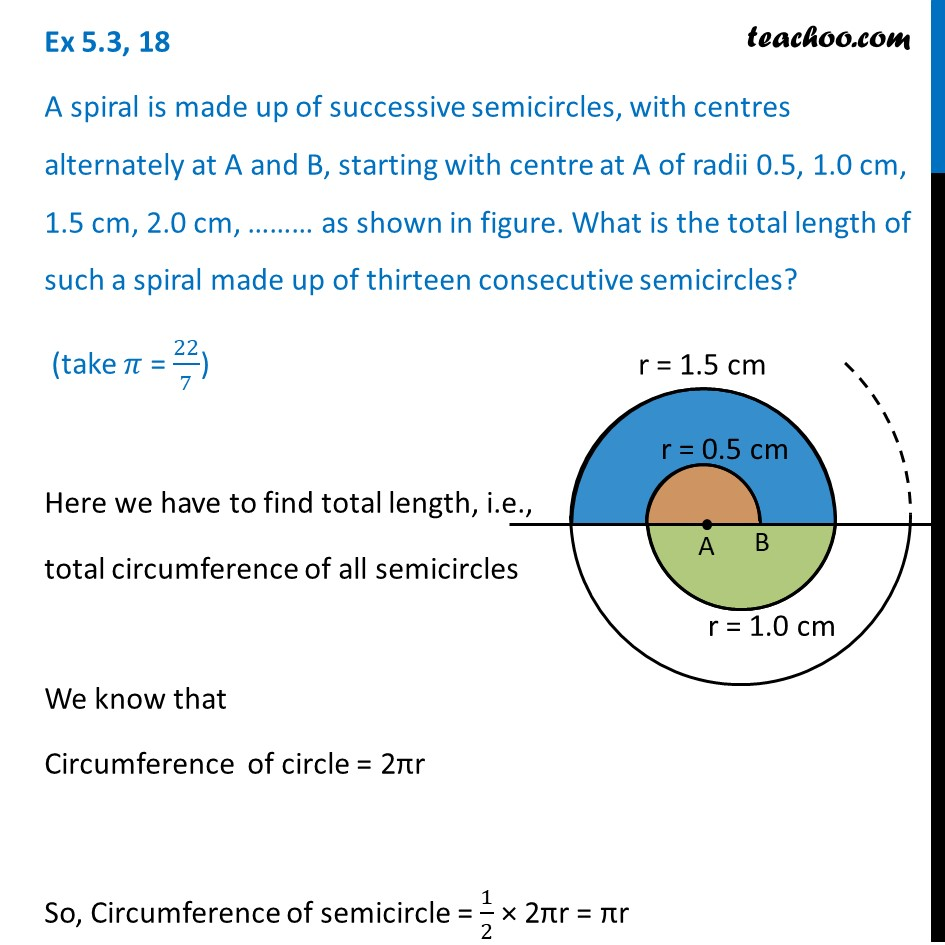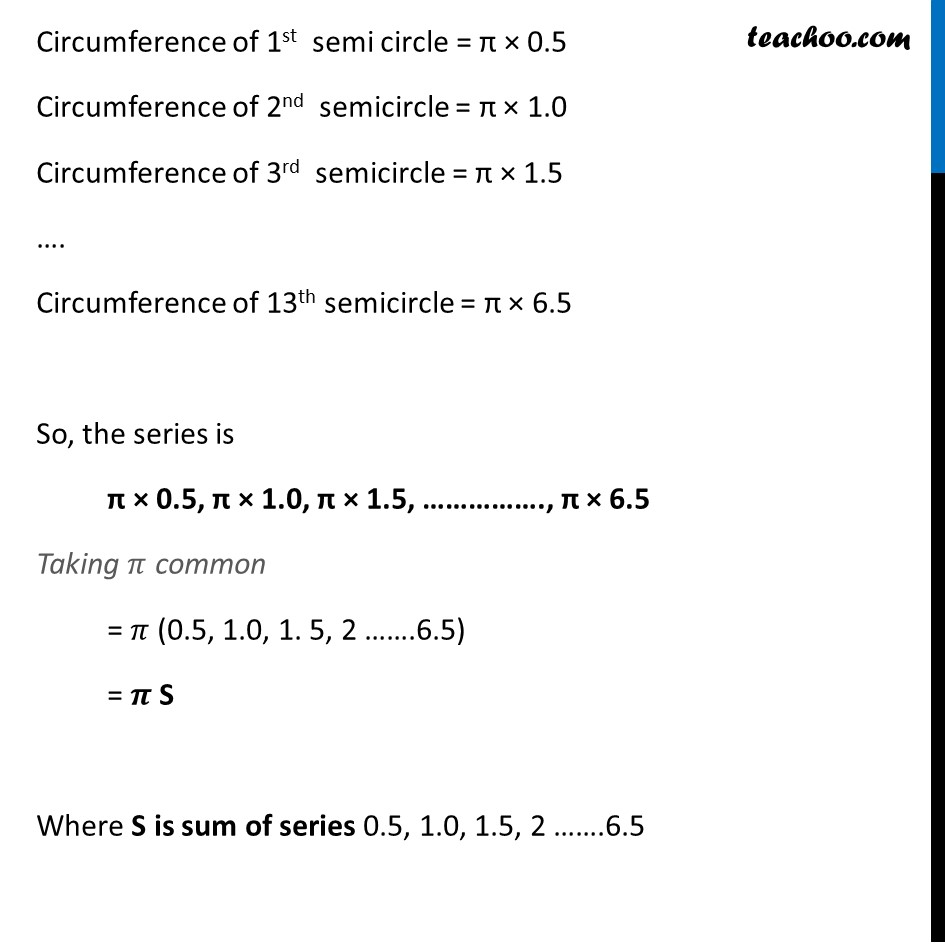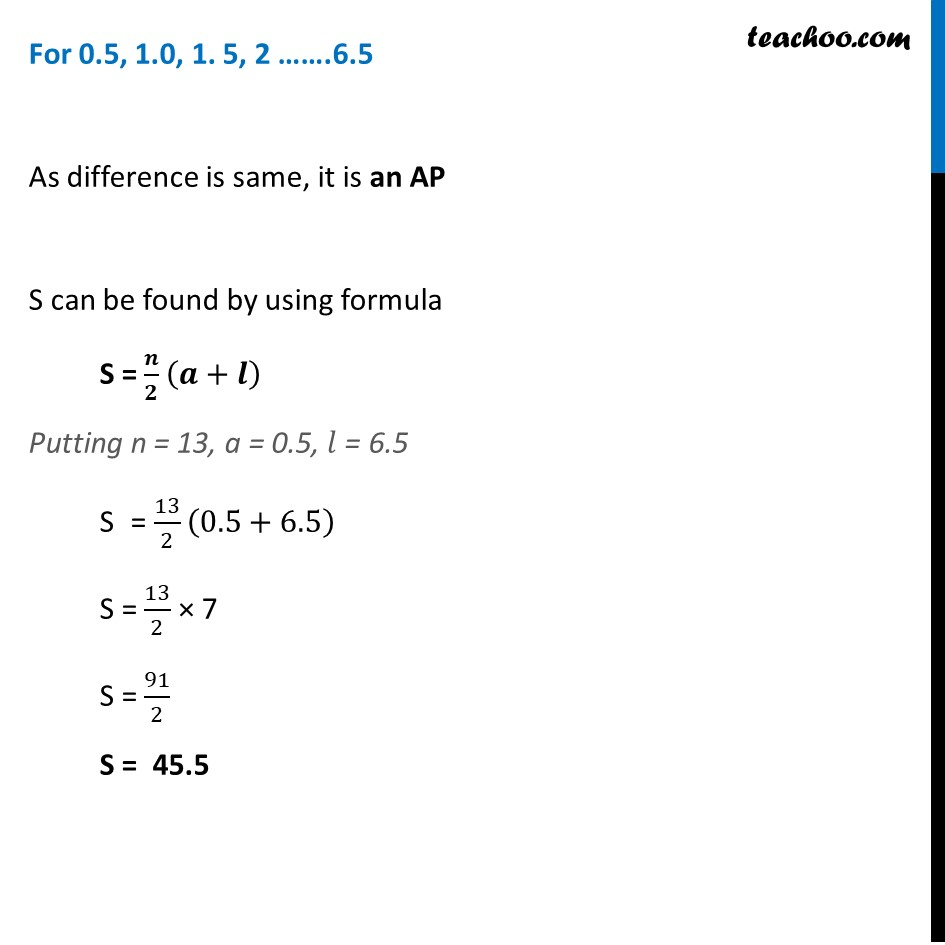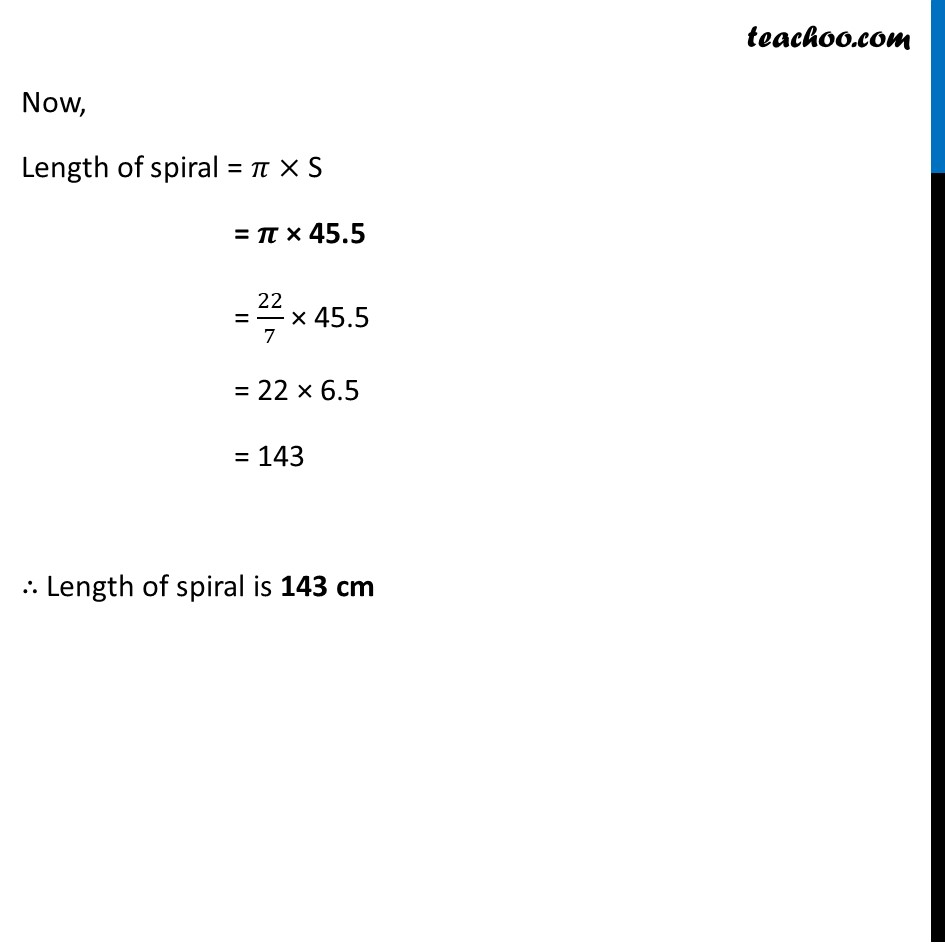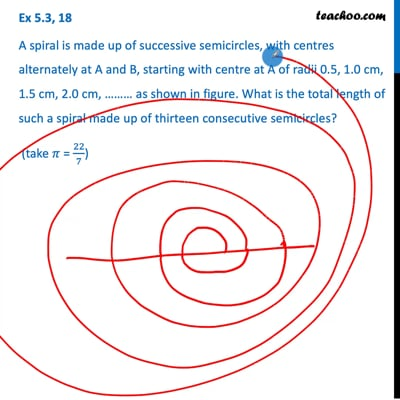This video is only available for Teachoo black users

### Transcript

Ex 5.3, 18 A spiral is made up of successive semicircles, with centres alternately at A and B, starting with centre at A of radii 0.5, 1.0 cm, 1.5 cm, 2.0 cm, ……… as shown in figure. What is the total length of such a spiral made up of thirteen consecutive semicircles? (take 𝜋 = 22/7) Here we have to find total length, i.e., total circumference of all semicircles We know that Circumference of circle = 2πr So, Circumference of semicircle = 1/2 × 2πr = πr Circumference of 1st semi circle = π × 0.5 Circumference of 2nd semicircle = π × 1.0 Circumference of 3rd semicircle = π × 1.5 …. Circumference of 13th semicircle = π × 6.5 So, the series is π × 0.5, π × 1.0, π × 1.5, ……………., π × 6.5 Taking 𝜋 common = 𝜋 (0.5, 1.0, 1. 5, 2 …….6.5) = 𝝅 S Where S is sum of series 0.5, 1.0, 1.5, 2 …….6.5 For 0.5, 1.0, 1. 5, 2 …….6.5 As difference is same, it is an AP S can be found by using formula S = 𝒏/𝟐 (𝒂+𝒍) Putting n = 13, a = 0.5, 𝑙 = 6.5 S = 13/2 (0.5+6.5) S = 13/2 × 7 S = 91/2 S = 45.5 Now, Length of spiral = 𝜋 × S = 𝝅 × 45.5 = 22/7 × 45.5 = 22 × 6.5 = 143 ∴ Length of spiral is 143 cm